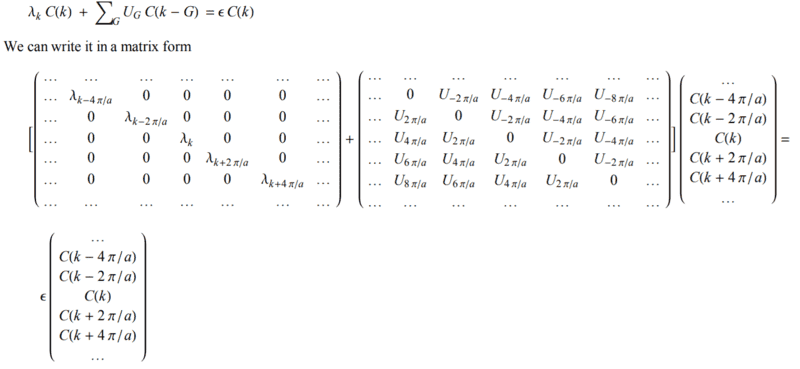# Central Equation Derivation: NxN Matrix?

• A
• semc
That's why people say it is a NxN matrix.In summary, the conversation discusses the derivation of the central equation, which is a set of equations for a fixed k in the first Brillouin zone. The equation couples coefficients Ck, Ck-G, Ck-G', Ck-G'', etc. and is represented as a NxN matrix. The conversation also mentions relevant resources for further understanding the equation.

#### semc

I went through the derivation for the central equation

$$(\lambda_k - \epsilon)C_k + \Sigma_G U_G C_{k-G} = 0$$

and everywhere I look everybody just says this is a NxN matrix. I don't see how this is true. Isn't this just a one line equation with summation across all reciprocal lattice? I don't understand why at one value of k we get NxN matrix. Any help is greatly appreciated!

Yeah this is what I found but the central equation is
$$\frac{\hbar^2k^2}{2m} C_k + \sum_{G}^{} V_GC_{k-G}=EC_k$$
so shouldn't I just have
$$\frac{\hbar^2k^2}{2m} C_k + V_0C_{k-g} + V_0C_{k+g}=EC_k$$
for G=ng and $$V=V_0e^{igx} +V_0e^{-igx} ?$$
How do you get the other equations
$$\frac{\hbar^2(k-g)^2}{2m} C_{k-g} + V_0C_{k-2g} + V_0C_{k}=EC_{k-g}$$

The "central equation" is a set of equations. For a fixed k in the first Brillouin zone, this set of equations for all reciprocal lattice vectors G couples those coefficients Ck , CkG , CkG' , CkG'' ,... whose wave vector differ from k by a reciprocal vector. Equation (107) in  which follows the presentation in the textbook “Solid State Physics” by Neil W. Ashcroft and N. David Mermin should illustrate the meaning of the "central equation". Have a look at , too.

 [PDF]Introduction to Solid State Physics
 [PDF]7.6 The Schrodinger equation of electron in a periodic potential

Last edited:
Well I read the book by ashcroft and mermin and  before. Everybody just says that the C(k) couples to C(k+G). Are they referring to the coupling via V0? I really don't see where the other equations come from

I guess I am asking why is C(k) a vector? Isn't C(k) just the Fourier coefficient of the wavefunction?#### Attachments

semc said:
I guess I am asking why is C(k) a vector?

C(k) isn't a vector. You simply write the set of equations which have to be solved in a matrix form.

Last edited: